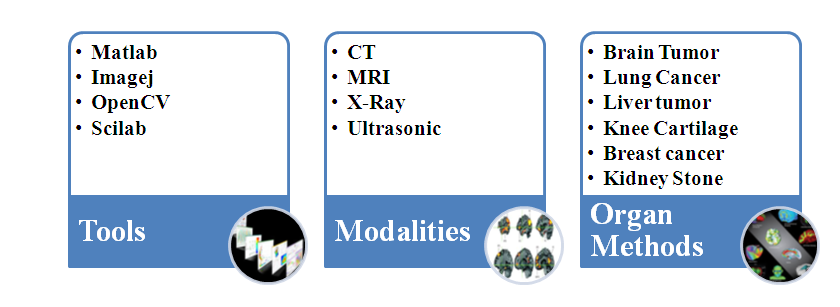# BIO MEDICAL MATLAB PROJECTS

Biomedical Matlab projects are developed for engineering students to submit their academic projects Biomedical projects are implemented using matlab simulation tool.

Biomedical Areas:

Domain in which Bio medical matlab projects can be carried out successfully.

• Tissue Engineering.
• Genetic Engineering.
• Neural Engineering.
• Clinical Engineering.
• BIO MEDICAL MATLAB PROJECTS 76%
• BIO MEDICAL MATLAB PROJECTS 24%

## Why to choose Bio Medical Projects using Matlab Tool?

Matlab have lots of tools used for building applications with custom graphical interfaces. Matlab functions are used to integrate Matlab algorithms with external applications and programming languages. Matlab provides range of numerical computation methods like as Differentiation and integration and Fourier analysis. Matlab functions are used to support one of the cardinal rules of programming.

Biomedical Concepts possible using matlab :

• Retinal Blood Vessel Segmentation
• Knee segmentation
• Brain Tissue Segmentation
• Lung Nodule Classification
• Kidney Stone Detection

Areas focused for Bio medical Matlab Projects:

• Cellular, tissue and genetic engineering.
• Bio instrumentation.
• Bio mechanics.
• Bio materials.### Sample Bio Medical Matlab Projects code for Brain Tissue Segmentation:-

Input =double(Input);
% imshow(Input,[]);
% pixval on[r c] = size(Input);
Length = r*c;
Dataset = reshape(Input,[Length,1]);
Clusters=3 %k CLUSTERS

Cluster1=zeros(Length,1);
Cluster2=zeros(Length,1);
Cluster3=zeros(Length,1);

% Initial Centroids
% ARBITARLY CHOOSE K OBJECTS AS INITIAL CENTROIDS
% miniv = min(min(Input));
% maxiv = max(max(Input));
% range = maxiv – miniv;
% stepv = range/Clusters;
% incrval = stepv;
% for i = 1:Clusters
% K(i).centroid = incrval;
% incrval = incrval + stepv;
% end
%
% no_of_cluster=3;
% ipimage=Input ;
% Test = InitCent(ipimage,no_of_cluster,0.75);
% for i = 1:no_of_cluster
% K(i).centroid = Test(i).Cent;
% end
%BEGIN LOOP
% L=1;

K(1).centroid =45
K(2).centroid =150
K(3).centroid =100

update1=0;
update2=0;
update3=0;
update4=0;
update5=0;

mean1=2;
mean2=2;
mean3=2;
mean4=2;
mean5=2;

while ((mean1 ~= update1) & (mean2 ~= update2) & (mean3 ~= update3) )

mean1=K(1).centroid;
mean2=K(2).centroid;
mean3=K(3).centroid;

for i=1:Length
for j = 1:Clusters
temp= Dataset(i);
difference(j) = abs(temp-K(j).centroid);

end[y,ind]=min(difference);

if ind==1
Cluster1(i) =temp;
end
if ind==2
Cluster2(i) =temp;
end
if ind==3
Cluster3(i) =temp;
end

end

%UPDATE CENTROIDS
cout1=0;
cout2=0;
cout3=0;

for i=1:Length